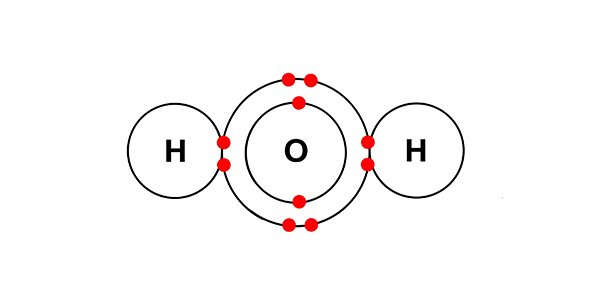# Electrolysis with different substances

## Example 2: Copper (II) chloride solution in water (where the electrodes are inert)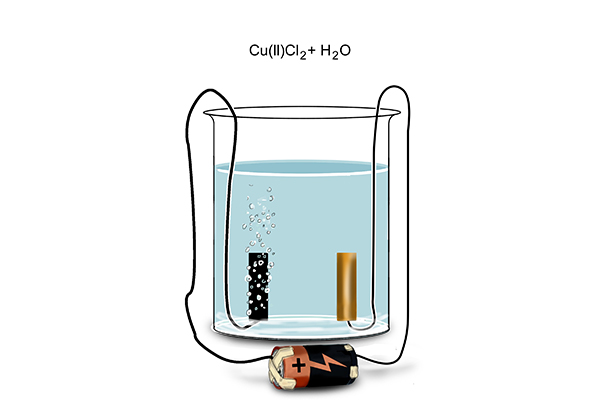If you are asked about the electrolysis of this solution in an exam you would not be expected to know the details of the shell arrangement for the element copper (because we only need the first 20 elements).

But the name 'copper (II)' is a massive clue and all you need to assume is that Cu(II) means Cu2+.

So starting with all the basic elements we get: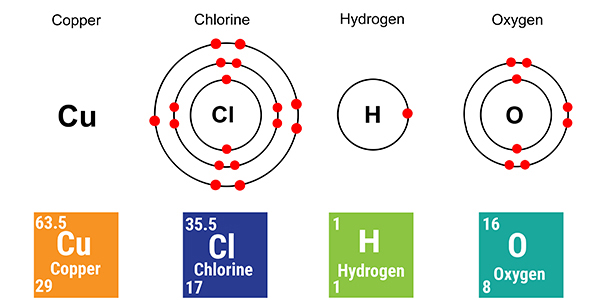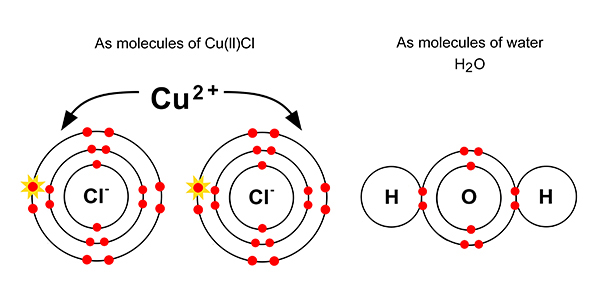But dissolved in solution with each other, chemists would say we have: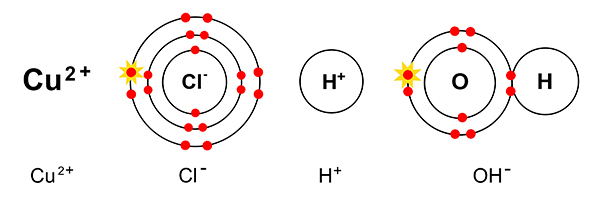###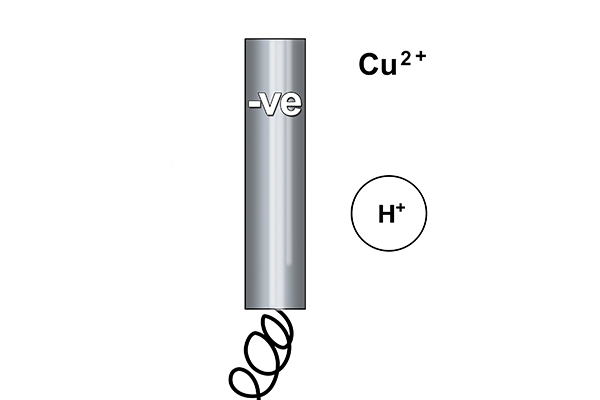Rule 1 says copper forms at the cathode not hydrogen (as copper is less reactive than hydrogen).

So at the cathode electrons are given out (to the copper ions) and: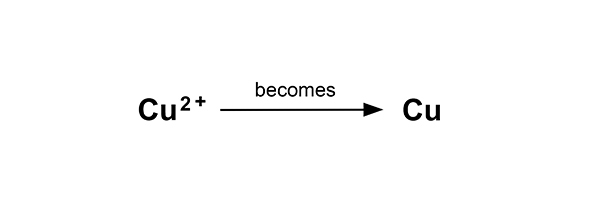This time, the pure copper begins to coat the electrode.

#### The half equation is:

Cu2+ (aq)          +          ?          →        Cu (s)

So the electron balance is      Cu2+                         +          2e-         →        Cu (s)

Or                                                Cu2+                                              →        Cu      –      2e-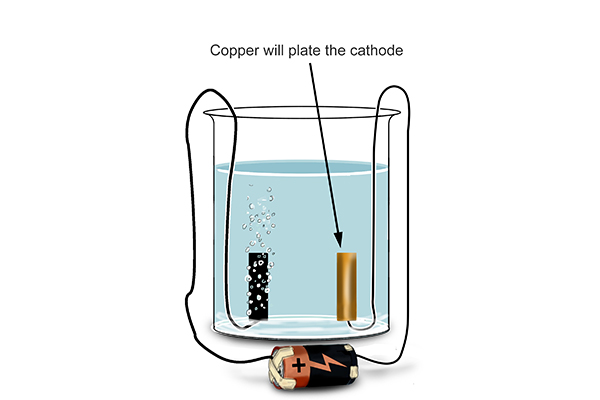### At the anode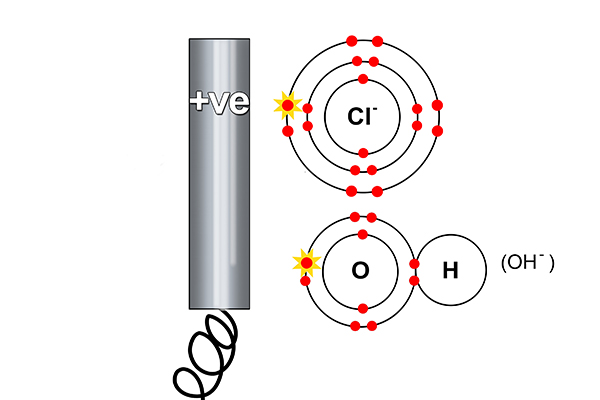Rule 2 says halogen (Cl) forms at the anode and not hydroxide (OH-). So at the anode, electrons are taken away from the chlorine.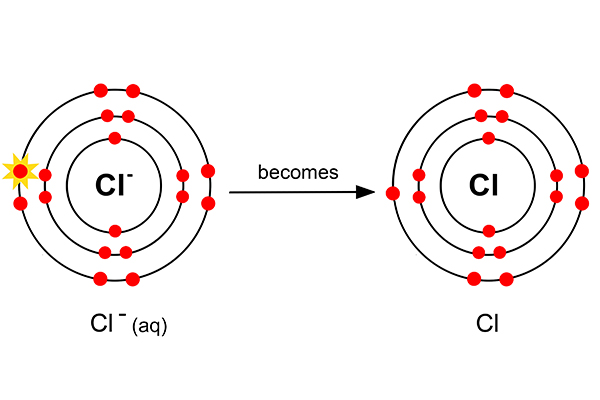But as chlorine always goes around in pairs (as in a diatomic gas), two chlorine atoms now combine to form chlorine gas.The half equation is:

Cl- (aq)             +          ?          →        Cl2 (g)

To balance this                        2Cl- (aq)           +          ?          →        Cl2 (g)

And the electron balance       2Cl- (aq)           -          2e-       →        Cl2 (g)

Or                                                2Cl-                                                         →        Cl2 + 2e-

The hydrogen and hydroxide ions left in the solution combine to form water.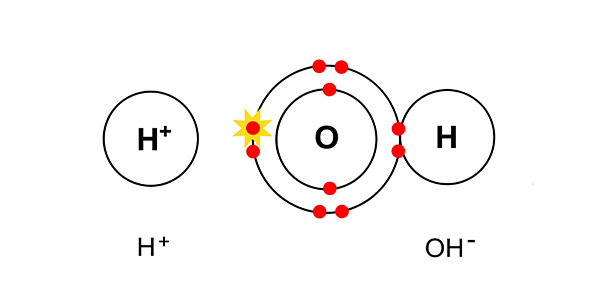They combine to give H2O.